# RS Aggarwal Solutions for Class 6 Maths Chapter 5 Fractions Exercise 5D

RS Aggarwal Solutions for Class 6 Maths Chapter 5 Exercise 5D are provided for students in PDF here. Questions related to Like and Unlike Fractions are available in Exercise 5D. Fractions having the same denominator are called Like Fractions and Fractions having different denominator are called Unlike Fractions. This exercise provides the students with knowledge about types of Fractions and methods of solving problems based on them. Students are advised to practice RS Aggarwal Solutions for Class 6 for better knowledge about the concepts which are covered under each exercise.

## Download PDF of RS Aggarwal Solutions for Class 6 Maths Chapter 5 Fractions Exercise 5D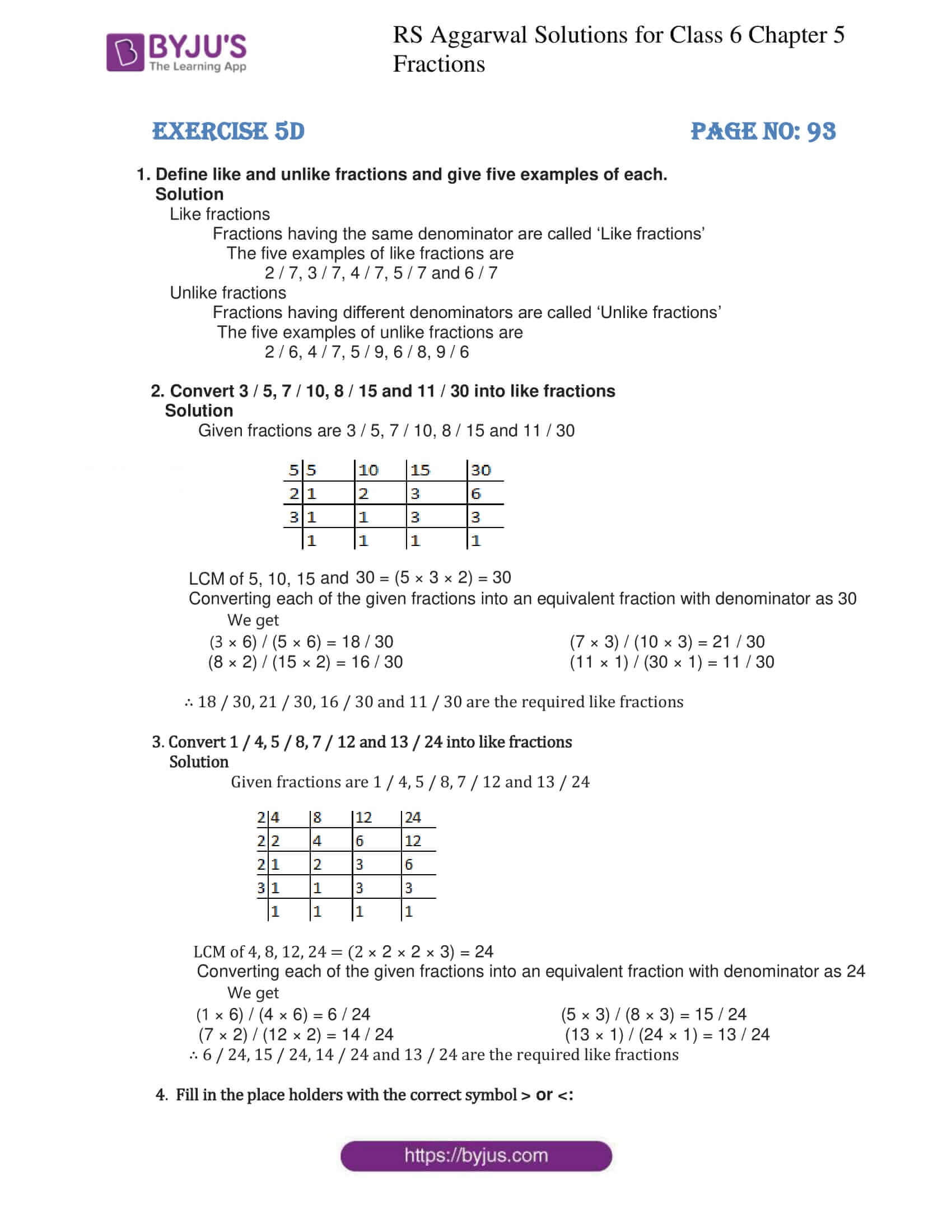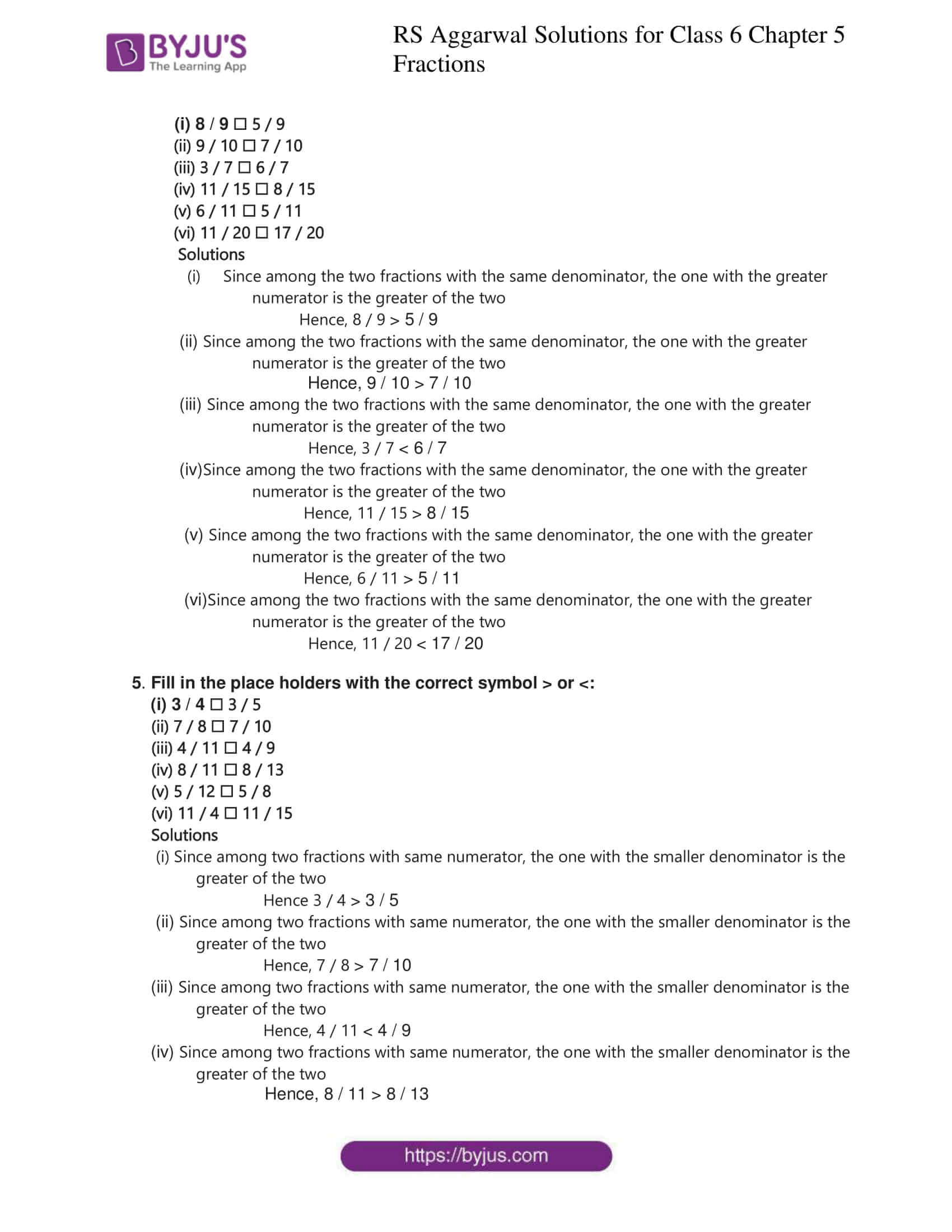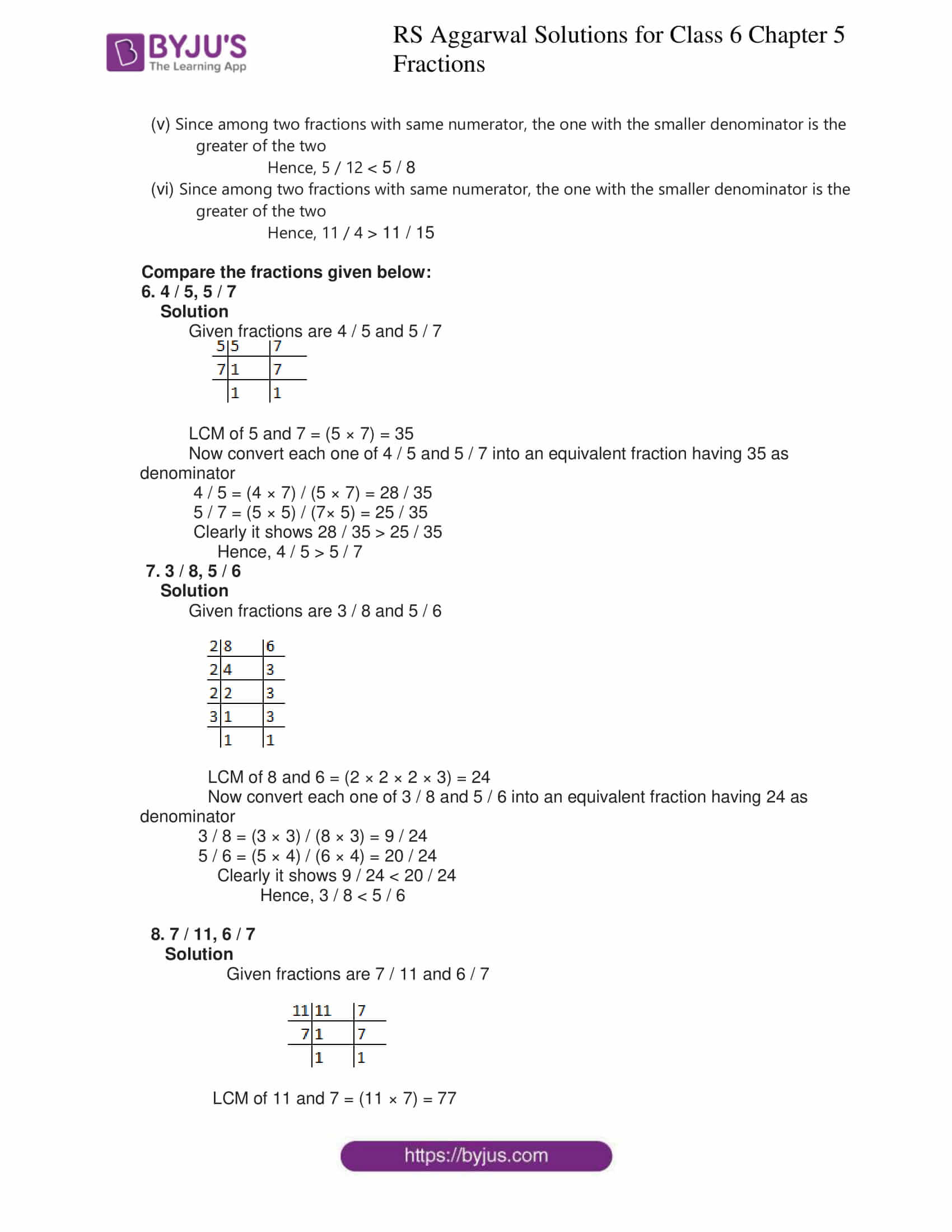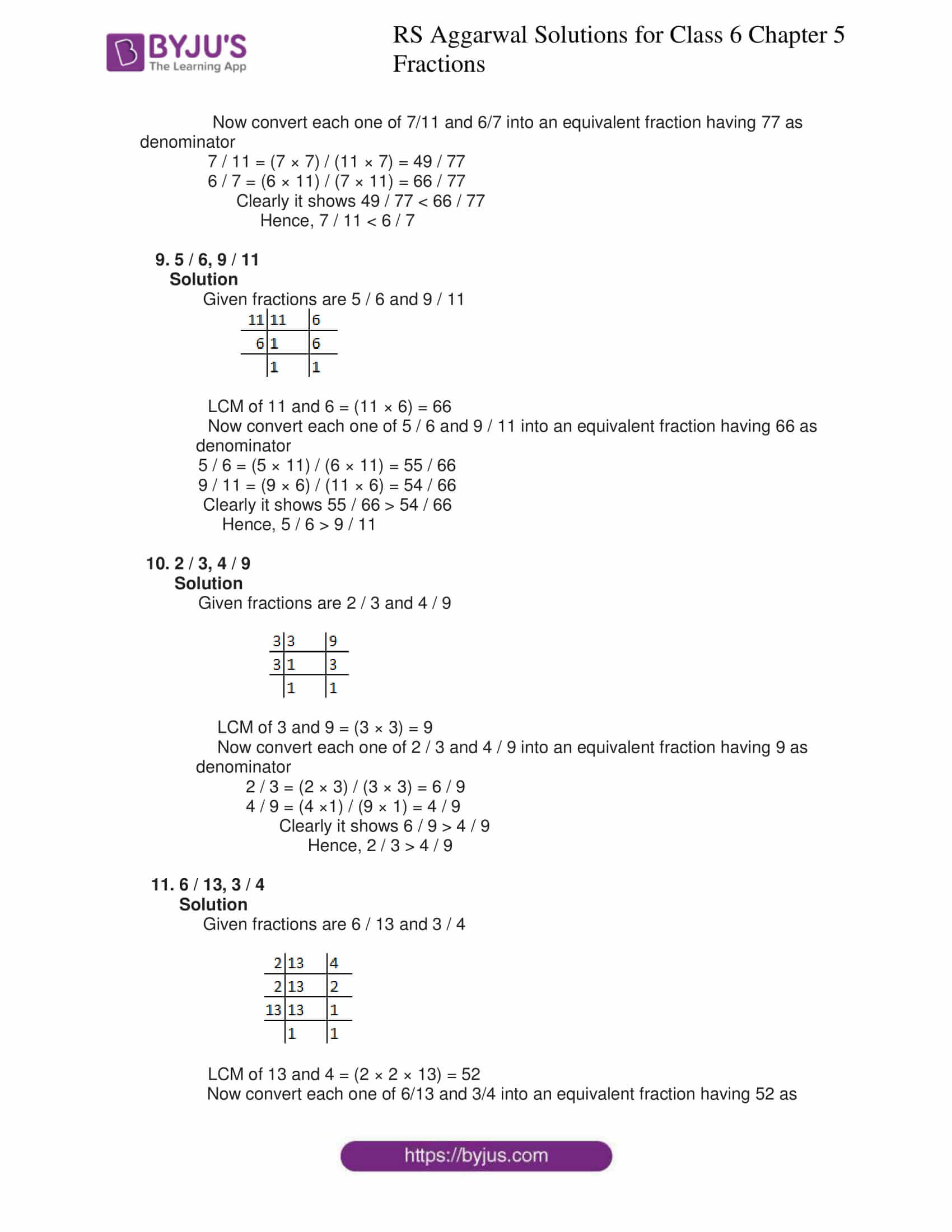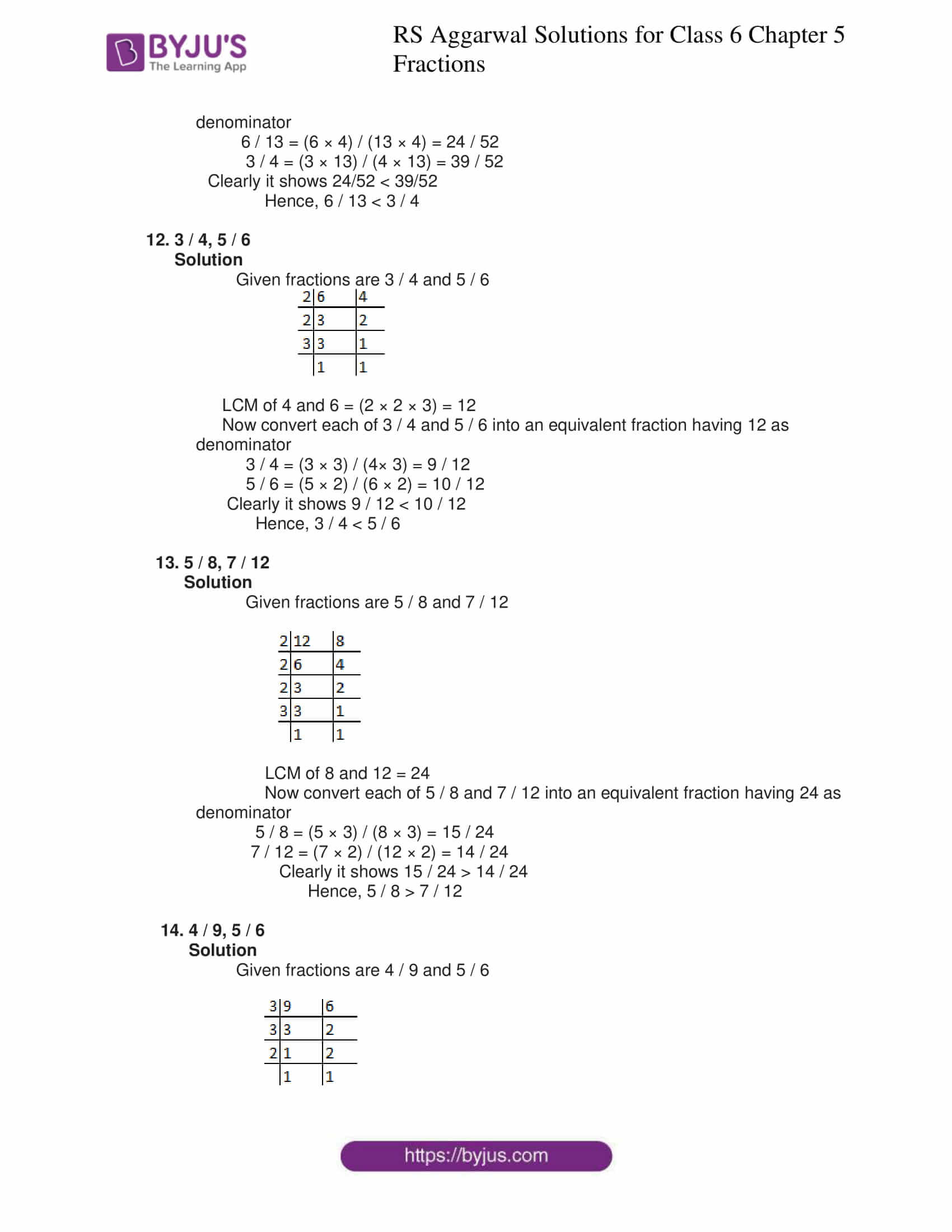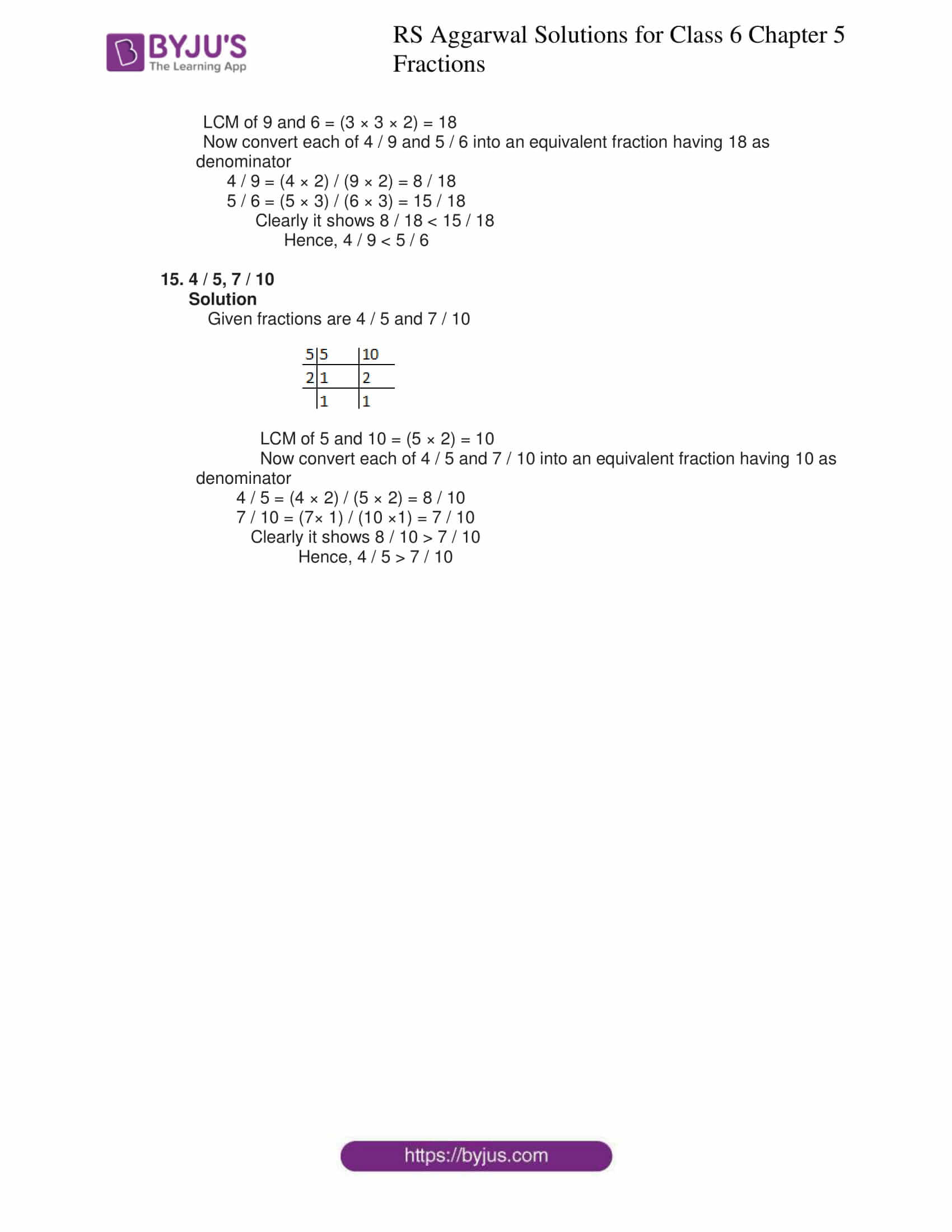### Access answers to Maths RS Aggarwal Solutions for Class 6 Chapter 5 Fractions Exercise 5D

1. Define like and unlike fractions and give five examples of each.

Solution

Like fractions

Fractions having the same denominator are called ‘Like fractions’

The five examples of like fractions are

2 / 7, 3 / 7, 4 / 7, 5 / 7 and 6 / 7

Unlike fractions

Fractions having different denominators are called ‘Unlike fractions’

The five examples of unlike fractions are

2 / 6, 4 / 7, 5 / 9, 6 / 8, 9 / 6

2. Convert 3 / 5, 7 / 10, 8 / 15 and 11 / 30 into like fractions

Solution

Given fractions are 3 / 5, 7 / 10, 8 / 15 and 11 / 30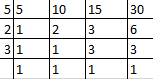LCM of 5, 10, 15 and 30 = (5 × 3 × 2) = 30

Converting each of the given fractions into an equivalent fraction with denominator as 30

We get

(3 × 6) / (5 × 6) = 18 / 30

(7 × 3) / (10 × 3) = 21 / 30

(8 × 2) / (15 × 2) = 16 / 30

(11 × 1) / (30 × 1) = 11 / 30

∴ 18 / 30, 21 / 30, 16 / 30 and 11 / 30 are the required like fractions

3. Convert 1 / 4, 5 / 8, 7 / 12 and 13 / 24 into like fractions

Solution

Given fractions are 1 / 4, 5 / 8, 7 / 12 and 13 / 24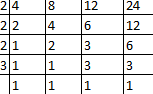LCM of 4, 8, 12, 24 = (2 × 2 × 2 × 3) = 24

Converting each of the given fractions into an equivalent fraction with denominator as 24

We get

(1 × 6) / (4 × 6) = 6 / 24

(5 × 3) / (8 × 3) = 15 / 24

(7 × 2) / (12 × 2) = 14 / 24

(13 × 1) / (24 × 1) = 13 / 24

∴ 6 / 24, 15 / 24, 14 / 24 and 13 / 24 are the required like fractions

4. Fill in the place holders with the correct symbol > or <:

(i) 8 / 9 ☐ 5 / 9

(ii) 9 / 10 ☐ 7 / 10

(iii) 3 / 7 ☐ 6 / 7

(iv) 11 / 15 ☐ 8 / 15

(v) 6 / 11 ☐ 5 / 11

(vi) 11 / 20 ☐ 17 / 20

Solutions

(i) Since among the two fractions with the same denominator, the one with the greater

numerator is the greater of the two

Hence, 8 / 9 > 5 / 9

(ii) Since among the two fractions with the same denominator, the one with the greater

numerator is the greater of the two

Hence, 9 / 10 > 7 / 10

(iii) Since among the two fractions with the same denominator, the one with the greater

numerator is the greater of the two

Hence, 3 / 7 < 6 / 7

(iv)Since among the two fractions with the same denominator, the one with the greater

numerator is the greater of the two

Hence, 11 / 15 > 8 / 15

(v) Since among the two fractions with the same denominator, the one with the greater

numerator is the greater of the two

Hence, 6 / 11 > 5 / 11

(vi)Since among the two fractions with the same denominator, the one with the greater

numerator is the greater of the two

Hence, 11 / 20 < 17 / 20

5. Fill in the place holders with the correct symbol > or <:

(i) 3 / 4 ☐ 3 / 5

(ii) 7 / 8 ☐ 7 / 10

(iii) 4 / 11 ☐ 4 / 9

(iv) 8 / 11 ☐ 8 / 13

(v) 5 / 12 ☐ 5 / 8

(vi) 11 / 4 ☐ 11 / 15

Solutions

(i) Since among two fractions with same numerator, the one with the smaller denominator is the

greater of the two

Hence 3 / 4 > 3 / 5

(ii) Since among two fractions with same numerator, the one with the smaller denominator is the

greater of the two

Hence, 7 / 8 > 7 / 10

(iii) Since among two fractions with same numerator, the one with the smaller denominator is the

greater of the two

Hence, 4 / 11 < 4 / 9

(iv) Since among two fractions with same numerator, the one with the smaller denominator is the

greater of the two

Hence, 8 / 11 > 8 / 13

(v) Since among two fractions with same numerator, the one with the smaller denominator is the

greater of the two

Hence, 5 / 12 < 5 / 8

(vi) Since among two fractions with same numerator, the one with the smaller denominator is the

greater of the two

Hence, 11 / 4 > 11 / 15

Compare the fractions given below:

6. 4 / 5, 5 / 7

Solution

Given fractions are 4 / 5 and 5 / 7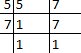LCM of 5 and 7 = (5 × 7) = 35

Now convert each one of 4 / 5 and 5 / 7 into an equivalent fraction having 35 as denominator

4 / 5 = (4 × 7) / (5 × 7) = 28 / 35

5 / 7 = (5 × 5) / (7× 5) = 25 / 35

Clearly it shows 28 / 35 > 25 / 35

Hence, 4 / 5 > 5 / 7

7. 3 / 8, 5 / 6

Solution

Given fractions are 3 / 8 and 5 / 6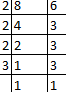LCM of 8 and 6 = (2 × 2 × 2 × 3) = 24

Now convert each one of 3 / 8 and 5 / 6 into an equivalent fraction having 24 as denominator

3 / 8 = (3 × 3) / (8 × 3) = 9 / 24

5 / 6 = (5 × 4) / (6 × 4) = 20 / 24

Clearly it shows 9 / 24 < 20 / 24

Hence, 3 / 8 < 5 / 6

8. 7 / 11, 6 / 7

Solution

Given fractions are 7 / 11 and 6 / 7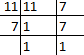LCM of 11 and 7 = (11 × 7) = 77

Now convert each one of 7/11 and 6/7 into an equivalent fraction having 77 as denominator

7 / 11 = (7 × 7) / (11 × 7) = 49 / 77

6 / 7 = (6 × 11) / (7 × 11) = 66 / 77

Clearly it shows 49 / 77 < 66 / 77

Hence, 7 / 11 < 6 / 7

9. 5 / 6, 9 / 11

Solution

Given fractions are 5 / 6 and 9 / 11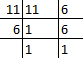LCM of 11 and 6 = (11 × 6) = 66

Now convert each one of 5 / 6 and 9 / 11 into an equivalent fraction having 66 as

denominator

5 / 6 = (5 × 11) / (6 × 11) = 55 / 66

9 / 11 = (9 × 6) / (11 × 6) = 54 / 66

Clearly it shows 55 / 66 > 54 / 66

Hence, 5 / 6 > 9 / 11

10. 2 / 3, 4 / 9

Solution

Given fractions are 2 / 3 and 4 / 9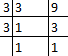LCM of 3 and 9 = (3 × 3) = 9

Now convert each one of 2 / 3 and 4 / 9 into an equivalent fraction having 9 as

denominator

2 / 3 = (2 × 3) / (3 × 3) = 6 / 9

4 / 9 = (4 ×1) / (9 × 1) = 4 / 9

Clearly it shows 6 / 9 > 4 / 9

Hence, 2 / 3 > 4 / 9

11. 6 / 13, 3 / 4

Solution

Given fractions are 6 / 13 and 3 / 4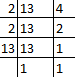LCM of 13 and 4 = (2 × 2 × 13) = 52

Now convert each one of 6/13 and 3/4 into an equivalent fraction having 52 as denominator

6 / 13 = (6 × 4) / (13 × 4) = 24 / 52

3 / 4 = (3 × 13) / (4 × 13) = 39 / 52

Clearly it shows 24/52 < 39/52

Hence, 6 / 13 < 3 / 4

12. 3 / 4, 5 / 6

Solution

Given fractions are 3 / 4 and 5 / 6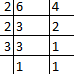LCM of 4 and 6 = (2 × 2 × 3) = 12

Now convert each of 3 / 4 and 5 / 6 into an equivalent fraction having 12 as

denominator

3 / 4 = (3 × 3) / (4× 3) = 9 / 12

5 / 6 = (5 × 2) / (6 × 2) = 10 / 12

Clearly it shows 9 / 12 < 10 / 12

Hence, 3 / 4 < 5 / 6

13. 5 / 8, 7 / 12

Solution

Given fractions are 5 / 8 and 7 / 12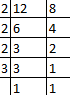LCM of 8 and 12 = 24

Now convert each of 5 / 8 and 7 / 12 into an equivalent fraction having 24 as

denominator

5 / 8 = (5 × 3) / (8 × 3) = 15 / 24

7 / 12 = (7 × 2) / (12 × 2) = 14 / 24

Clearly it shows 15 / 24 > 14 / 24

Hence, 5 / 8 > 7 / 12

14. 4 / 9, 5 / 6

Solution

Given fractions are 4 / 9 and 5 / 6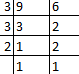LCM of 9 and 6 = (3 × 3 × 2) = 18

Now convert each of 4 / 9 and 5 / 6 into an equivalent fraction having 18 as

denominator

4 / 9 = (4 × 2) / (9 × 2) = 8 / 18

5 / 6 = (5 × 3) / (6 × 3) = 15 / 18

Clearly it shows 8 / 18 < 15 / 18

Hence, 4 / 9 < 5 / 6

15. 4 / 5, 7 / 10

Solution

Given fractions are 4 / 5 and 7 / 10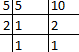LCM of 5 and 10 = (5 × 2) = 10

Now convert each of 4 / 5 and 7 / 10 into an equivalent fraction having 10 as

denominator

4 / 5 = (4 × 2) / (5 × 2) = 8 / 10

7 / 10 = (7× 1) / (10 ×1) = 7 / 10

Clearly it shows 8 / 10 > 7 / 10

Hence, 4 / 5 > 7 / 10